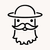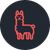# Need some assistance with an If statement

206
1
08-31-2022 06:41 AMOccasional Contributor III

I am trying to evaluate the possible answers of previous select_one questions and calculate a 'Yes' or 'No' into a final question.

if 'pro_license' = 'No' and 'front_end_complete = 'Yes' then 'registration_complete' should be 'Yes'

OR

if 'pro_license' = 'Yes' AND 'front_end_complete = 'Yes' AND 'backend_end_complete = 'Yes' then 'registration_complete should be 'Yes'

Can someone help consolidate this logic into a single expression?

Here is what I've tried, I'm sure there are several issues here but I'm struggling to figure this out.

``if(selected(\${pro_license}, 'No') and selected(\${front_end_complete}), 'Yes', 'Yes', 'No'), if(selected(\${pro_license}, 'Yes') and selected(\${front_end_complete}, 'Yes') and selected(\${back_end_complete}, 'Yes', 'Yes', 'No'))``

1 Solution

Accepted SolutionsbyEsri Contributor

With If statements the syntax is what you're evaluating, the answer if true, and the answer if false.

If we look at the first half of your calculation:

``if(selected(\${pro_license}, 'No') and selected(\${front_end_complete}), 'Yes', 'Yes', 'No'),``

What you have here is if your evaluation is false give an answer of no, but that is not what you want. What you actually want is if the answer is false, then continue and check the following evaluation to see if it is true. Only after all of your chained evaluations return false do you want to provide the 'No'.

``````if(\${pro_license}='No' and \${front_end_complete}='Yes', 'Yes',
if(\${pro_license}='Yes' and \${front_end_complete}='Yes' and \${back_end_complete}='Yes', 'Yes','No'))``````

- JenbyEsri Contributor

With If statements the syntax is what you're evaluating, the answer if true, and the answer if false.

If we look at the first half of your calculation:

``if(selected(\${pro_license}, 'No') and selected(\${front_end_complete}), 'Yes', 'Yes', 'No'),``

What you have here is if your evaluation is false give an answer of no, but that is not what you want. What you actually want is if the answer is false, then continue and check the following evaluation to see if it is true. Only after all of your chained evaluations return false do you want to provide the 'No'.

``````if(\${pro_license}='No' and \${front_end_complete}='Yes', 'Yes',
if(\${pro_license}='Yes' and \${front_end_complete}='Yes' and \${back_end_complete}='Yes', 'Yes','No'))``````

- Jen